Documentation

## PDSCH Throughput Performance in Simulink

This example demonstrates how to measure the physical downlink shared channel (PDSCH) throughput performance in Simulink® using LTE Toolbox™ for the following transmission modes (TM):

• TM1: Single antenna (Port 0)

• TM2: Transmit diversity

• TM3: Open loop codebook based precoding: Cyclic Delay Diversity (CDD)

### Introduction

The model in this example measures the throughput of an end-to-end PDSCH simulation. The Simulink model can operate under TM1, TM2 and TM3 transmission modes without implementing hybrid automatic repeat request (Hybrid ARQ). For an equivalent simulation in MATLAB®, see the example PDSCH Throughput Conformance Test for Single Antenna (TM1), Transmit Diversity (TM2), Open Loop (TM3) and Closed Loop (TM4/6) Spatial Multiplexing, which additionally covers TM4/6 and enables Hybrid ARQ. For information on modeling TM7, TM8, TM9 and TM10, see the following MATLAB example: PDSCH Throughput for Non-Codebook Based Precoding Schemes: Port 5 (TM7), Port 7 or 8 or Port 7-8 (TM8), Port 7-14 (TM9 and TM10).

The example works on a subframe by subframe basis. For each subframe, the model generates and OFDM modulates a populated resource grid to create a transmit waveform. The generated waveform is then passed through a noisy fading channel. The receiver then performs channel estimation, equalization, demodulation, and decoding. The block CRC result at the output of the channel decoder is used to determine the throughput performance of the PDSCH.

The MATLAB Function block enables the use of MATLAB functions in a Simulink model. In this example, the end-to-end simulation is modeled in Simulink by using MATLAB Function blocks to call LTE Toolbox functions. The coder.extrinsic construct is used at the top of every MATLAB Function block to declare the function extrinsic during simulation. This construct enables you to call MATLAB functions in Simulink that do not support code generation.

### Structure of the Model

The model has four main parts:

• Transmitter: Generates random codewords and a populated resource grid, which is OFDM modulated to create a transmit waveform.

• Channel: Filters the transmitted waveform through a multipath Rayleigh fading channel with AWGN.

• Receiver: Recovers the transmitted sequence of bits by performing synchronization, channel estimation, equalization, demodulation, and decoding.

• Throughput analysis: Calculates the throughput performance with the block CRC decoding result.

Finally, the Model Parameters block enables you to vary the most common parameters for the simulation, channel modeling, and channel estimation.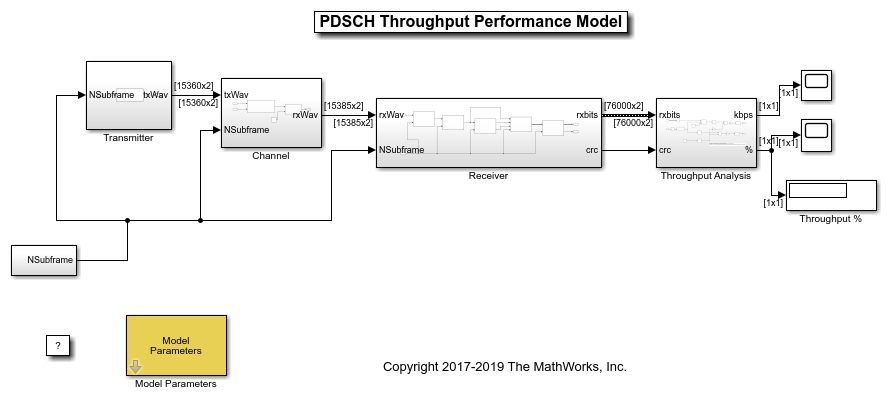### Transmitter

The Transmitter block creates one or two random codewords with information bits, depending on the transmission mode. Then the call to the lteRMCDLTool function produces an OFDM modulated waveform from the information bits. This waveform contains the physical channels and signals. Since the model does not specify an RMC, all downlink subframes are scheduled.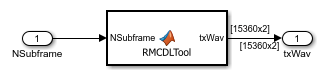### Channel

In the channel subsystem, the waveform is passed through a multipath Rayleigh fading channel and AWGN noise is added. The noise power is controlled by varying the signal to noise ratio (SNR) parameter. The parameters of the fading process can be controlled from the Channel Parameters tab in the Model Parameters block.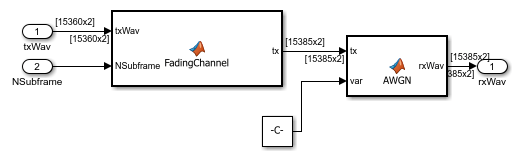### Receiver

The receiver recovers the PDSCH data from the channel and computes the block CRC. This process consists of the following steps:

1. Synchronization: The received symbols are offset to account for a combination of implementation delay and channel delay spread.

2. OFDM Demodulation: The received symbols are OFDM demodulated.

3. Channel Estimation: The channel response and noise levels are estimated. These estimates are used to decode the PDSCH.

4. PDSCH Decoding: The recovered PDSCH symbols for each transmit antenna from the received grid, along with a channel estimate, are demodulated and descrambled to obtain an estimate of the received codewords.

5. Downlink Shared Channel (DL-SCH) decoding and block CRC error computing: The vector of decoded soft bits is passed to lteDLSCHDecode. This function decodes the codeword and returns the block CRC error used to determine the throughput of the system.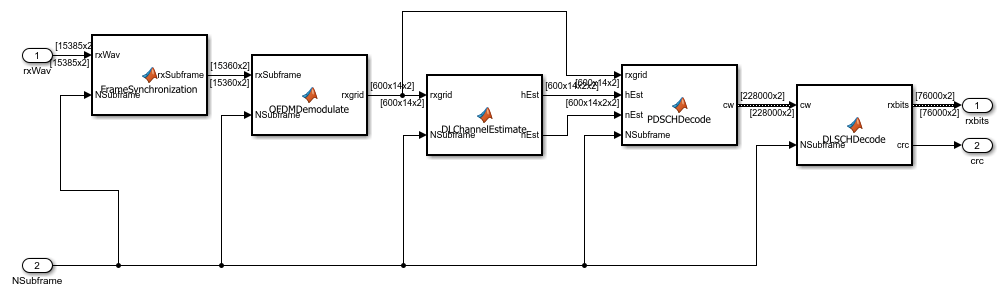### Throughput Analysis

In this block, the throughput performance of the link is calculated, both in kbps and in percentage, by using the block CRC result from the receiver.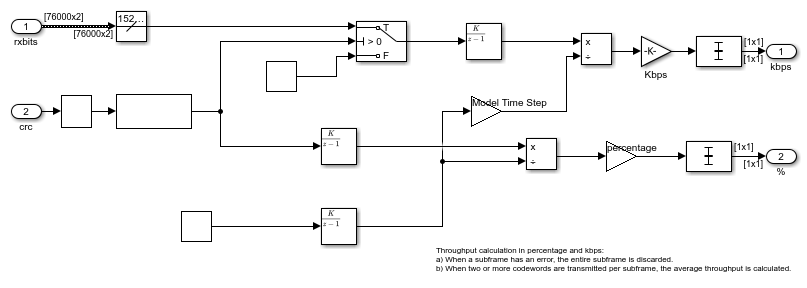### Results and Displays

When the simulation is run, the throughput per codeword is displayed both in percentage and in kbps. To obtain representative results, run the simulation long enough for the throughput results to reach a steady state. By default, 20 frames (0.2 s of simulation time) are simulated.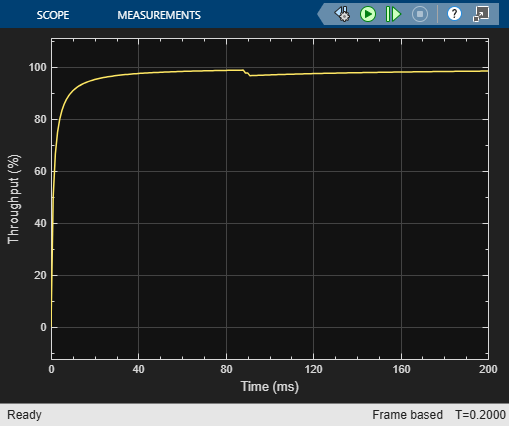### Exploring the Example

Try changing the SNR and the transmission mode in the Model Parameters block. Decreasing the SNR will decrease the throughput of the system since more subframes will be lost. Simulating different TMs will result in different throughput in kbps and percentage for the same SNR.

You can also try changing several channel parameters, such as the number of receive antennas or the MIMO correlation. Other parameters to try changing include the channel estimator parameters, such as the window type and window size.